TitleIntermediate Algebra Tutorial 3: Sets of Numbers

WTAMU > Virtual Math Lab > Intermediate AlgebraLearning Objectives

 After completing this tutorial, you should be able to: Graph a point on a real number line. Know what a set and an element are. Indicate if something is an element or is not an element of a set, using the appropriate notation. Tell if one set is a subset of another set. Read or write out a set in either roster form or set builder form. Know what the empty set is and how to notate it. Identify what numbers belong to the set of natural numbers, whole numbers, integers, rational numbers, irrational numbers, and real numbers. Find the absolute value of a number. Find the opposite of a number.Introduction

 Have you ever sat in a math class, and you swear the teacher is speaking some foreign language?  Well, algebra does have it's own lingo.  This tutorial will go over some key definitions and phrases used when specifically working with sets of numbers as well as absolute values and opposites.  Even though it may not be the exciting part of math,  it is very important that you understand the language spoken in algebra class.  It will definitely help you do the math that comes later.  Of course, numbers are very important in math. This tutorial helps you to build an understanding of what the different sets of numbers are.   You will also learn what set(s) of numbers specific numbers,  like -3, 0, 100, and even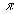(pi) belong to.  Some of them belong to more than one set.  I think you are ready to go forward.  Let's make you a numeric set whiz kid (or adult).Tutorial

 Real Number Line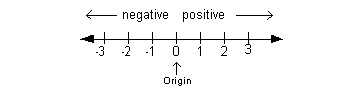Above is an illustration of a number line. Zero, on the number line, is called the origin.  It separates the negative numbers (located to the left of 0) from the positive numbers (located to the right of 0).  I feel sorry for 0, it does not belong to either group.  It is neither a positive or a negative number.     When graphing a point on the number line, you simply color in a point that corresponds to that number on the number line as illustrated below.  That is how you graph a solution on the number line.  This is how you would graph it if your solution was the number 2: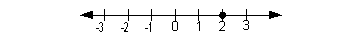Sets and Elements

 A set is a collection of objects.  Those objects are generally called elements of the set.  The symbol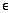means 'is an element of.' So, it stands to reason thatrepresents 'is not an element of.'

 Subset

 We say that A is a subset of B, written AB, when every element of A is contained in B. (It does not necessarily mean that every element of B is also contained in A)

 Ways to Notate Sets

 There are several ways to notate a set,  the two most common ways are: the roster form and  set builder notation.

 Roster form just lists out the elements of a set between two set brackets.  For example,   {January, June, July} Set builder notation describes the members of the set without listing them.  It is also written between two set brackets.    For example, {x | x is a month that begins with J} When writing it in set builder notation you always do the following: start off with a left set bracket, then you put x followed by a vertical bar which is interpreted as 'such that'. Then you write out the description of the elements of the set.  Finish it with a right set bracket. So the above illustration would be read: "x, such that, x is a month that begins with J."  It is important to know set builder notation, especially in mathematics, because it allows you to group together large number of elements that belong to a certain category.  The above set has only 3 elements, so it would not be difficult to write it in roster form as shown above. However,  if your set has hundreds or thousands of elements, it would be hard to list them out, but easy to refer to them using set builder notation.  For example, {x| x is a college student in Texas}.  Before we move on to the math aspect of sets, there is one more term we need to make sure you have a handle on.

 Empty Set

 Empty (or null) set is a set that contains no elements.  It is symbolized by {   }   OR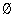. Be careful.  It is real tempting to use them together, but {} IS NOT a way to indicate empty set.       Let's move on to some special sets that pertain specifically to math. Note that the three dots shown in the sets below are called ellipsis.  It indicates that the elements in the set would continue in the same pattern. - In other words, the list would keep going and going in that direction using the pattern illustrated.

 Natural (or Counting) Numbers  N = {1, 2, 3, 4, 5, ...}

 Makes sense, we start counting with the number 1 and continue with 2, 3, 4, 5, and so on.

 Whole Numbers  {0, 1, 2, 3, 4, 5, ...}

 The only difference between this set and the one above is that this set not only contains all the natural numbers, but it also contains 0,  where as 0 is not an element of the set of natural numbers.

 Integers Z = {..., -5, -4, -3, -2, -1, 0, 1, 2, 3, 4, 5, ...}

 This set adds on the negative counterparts to the already existing whole numbers (which, remember, includes the number 0). The natural numbers and the whole numbers are both subsets of integers.

 Rational Numbers Q = {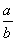| a and b are integers and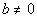}

 In other words, a rational number is a number that can be written as one integer over another. Be very careful. Remember that a whole number can be written as one integer over another integer. The integer in the denominator is 1 in that case. For example, 5 can be written as 5/1. The natural numbers, whole numbers, and integers are all subsets of rational numbers.

 Irrational Numbers I = {x | x is a real number that is not rational}

 In other words, an irrational number is a number that can not be written as one integer over another.  It is a non-repeating, non-terminating decimal. One big example of irrational numbers is roots of numbers that are not perfect roots - for example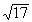or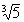. 17 is not a perfect square - the answer is a non-terminating, non-repeating decimal, which CANNOT be written as one integer over another.  Similarly, 5 is not a perfect cube. It's answer is also a non-terminating, non-repeating decimal. Another famous irrational number is(pi).  Even though it is more commonly known as 3.14, that is a rounded value for pi.  Actually it is 3.1415927... It would keep going and going and going without any real repetition or pattern. In other words, it would be a non terminating, non repeating decimal, which again, can not be written as a rational number, 1 integer over another integer.

 Real Numbers R = {x | x corresponds to point on the number line}

 Any number that belongs to either the rational numbers or irrational numbers would be considered a real number. That would include natural numbers, whole numbers and integers.Example 1:   List the elements of the set {x | x is a whole number less than 11}

 There are two parts to this: the number has to belong to the set of whole numbers {0, 1, 2, 3, ...}  and it has to be less than 11. Putting these two ideas together we get: {0, 1, 2, 3, ..., 9, 10}Example 2:  List the elements of the set {x | x is a natural number between 5 and 11}.

 There are two parts to this: the number has to belong to the set of natural numbers {1, 2, 3, ...}  and  it has to be between 5 and 11 - which by the way would not include those two numbers. Let's see what we get when we put those ideas together: {6, 7, 8, 9, 10} You would not have an ellipsis after the 10 because this set would stop at the number 10.Example 3:  List the elements of the set {x | x is a natural number greater than 100}.

 There are two parts to this: the number has to belong to the set of natural numbers {1, 2, 3, ...}  and  it has to be greater than 100. Let's see if you figured this one out ok: {101, 102, 103, 104, ...} Did you remember to include the ellipsis to show that the set would continue on and on in the same pattern?Example 4:   Graph the set on a number line.   {-2, -.5, 0, 1/5, 3}

 When you graph fractions or decimals, you need to first figure out between what two integers  it belongs and then estimate, depending on the fraction, where you are going to place the point between those two numbers. In this problem, we have a -.5, which is between -1 and 0.  Since it is halfway between these two numbers, I would place the dot halfway between. We also have the fraction 1/5, which is between 0 and 1 and since it is closer to 0 than 1, I would place it accordingly on the graph. The other numbers are integers that are already marked clearly on the graph. Let's see what we get when we graph all of these real numbers: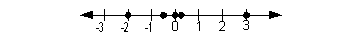Example 5:   List the elements of the following sets that are also elements of the given set {-4, 0, 2.5,,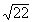,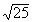, 11/2, 7} Natural numbers, whole numbers, integers, rational numbers, irrational numbers, and real numbers.

 Natural numbers? The numbers in the given set that are also natural numbers are {, 7}. Note thatsimplifies to be 5, which is a natural number.

 Whole numbers? The numbers in the given set that are also whole numbers are {0,, 7}.

 Integers? The numbers in the given set that are also integers are {-4, 0,, 7}.

 Rational numbers? The numbers in the given set that are also rational numbers are {-4, 0, 2.5,, 11/2, 7}.

 Irrational numbers? The numbers in the given set that are also irrational numbers are {,}. These two numbers CANNOT be written as one integer over another.  They are non-repeating, non-terminating decimals.

 Real numbers? The numbers in the given set that are also real numbers are {-4, 0, 2.5,,,, 11/2, 7}.Example 6:   Place aorto make the statement true.  0  ?  {x | x is a whole number}

 Since 0 is one of the elements listed in the set of whole numbers, then it would be true to say 0{x | x is a whole number}.Example 7:   Place aorto make the statement true.    -2  ?  {2, 4, 6, .... }

 Since -2 is not listed and the ellipsis would indicate listing out more positive even numbers, it looks like -2 is not part of this set.  Therefore, it would be a true statement to say -2{2, 4, 6, .... }.Example 8:   Place aorto make the statement true. ½  ? {x | x is an irrational number}

 Since ½ is written as one integer over another, it would be a rational number as opposed to an irrational number.  So the true statement would be ½{x | x is an irrational number}.Example 9:   Place aorto make the statement true.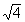?    {x | x is a rational number}

 Sincesimplifies to be 2, which can be written as 2/1, we can write the following true statement{x | x is a rational number}.Example 10:   Determine if the statement is true or false? NI

 Since not every element of N (natural numbers) is found in I (irrational numbers), then this statement is FALSE. In fact, there are no elements in N that are in I.Example 11:   Determine if the statement is true or false?IR

 Since EVERY element of I (Irrational numbers) is also in R (Real numbers), then this statement is TRUE.Example 12:   Determine if the statement is true or false? NQ

 Since EVERY element of N (Natural numbers) is also in Q (Rational numbers), then this statement is TRUE.

 Absolute Value

 Most people know that when you take the absolute value of ANY number (other than 0)  the answer is positive.  But, do you know WHY? Well, let me tell you why! The absolute value of x, notated |x|, measures the DISTANCE that x is away from the origin (0) on the real number line.  Aha!  Distance is always going to be positive (unless it is 0) whether the number you are taking the absolute value of is positive or negative.   The following are illustrations of what absolute value means using the numbers 3 and -3: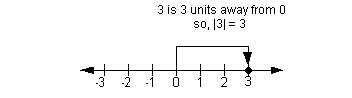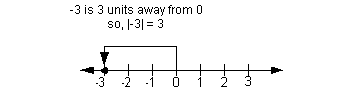Example 13:    Find the absolute value.    |-7|

 When looking for the absolute value of -7, we are looking for the number of units (or distance) -7 is from 0 on the number line. I came up with 7, how about you?Example 14:   Find the absolute value.    |7|

 When looking for the absolute value of 7, we are looking for the number of units (or distance) 7 is from 0 on the number line. I came up with 7, how about you?Example 15:   Find the absolute value.     - |-2|

 This problem has a little twist to it.  Let's talk it through.  First of all, if we just concentrate on |-2|, we would get 2.  Second,  note that there is a negative on the OUTSIDE of the absolute value.  That means we are going to take the opposite of what we get for the absolute value.  Putting that together we get -2 for our answer.   Note that the absolute value part of the problem was still positive.  We just had a negative on the outside of it that made the final answer negative.

 Opposites

 Opposites are two numbers that are on opposite sides of the origin (0) on the number line, but have the same absolute value.   In other words, opposites are the same distance away from the origin, but in opposite directions. The opposite of x is the number -x. Keep in mind that the opposite of 0 is 0.   The following is an illustration of opposites using the numbers 3 and -3: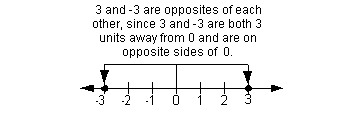Double Negative Property

 The double negative property reads that for every real number a, -(-a) = a. When you see a negative sign in front of an expression, you can think of it as taking the opposite of it.  For example, if you had -(-2), you can think of it as the opposite of -2.  Since a number can only have one of two signs, either a '+' or a '-', then the opposite of a negative would have to be positive.  So, -(-2) = 2.Example 16:   Write the opposite of 1.5.

 The opposite of 1.5 is -1.5, since both of these numbers have the same absolute value but are on opposite sides of the origin on the number line.Example 17:   Write the opposite of -3.

 The opposite of -3 is 3, since both of these numbers have the same absolute value but are on opposite sides of the origin on the number line.Practice Problems

 These are practice problems to help bring you to the next level.  It will allow you to check and see if you have an understanding of these types of problems. Math works just like anything else, if you want to get good at it, then you need to practice it.  Even the best athletes and musicians had help along the way and lots of practice, practice, practice, to get good at their sport or instrument.  In fact there is no such thing as too much practice. To get the most out of these, you should work the problem out on your own and then check your answer by clicking on the link for the answer/discussion for that  problem.  At the link you will find the answer as well as any steps that went into finding that answer.Practice Problems 1a - 1c: List the elements of each set.

 1a. {x | x is a natural number less than 4} (answer/discussion to 1a)

 1b. {x | x is a whole number between 100 and 105} (answer/discussion to 1b)

 1c. {x | x is an odd whole number greater than 5} (answer/discussion to 1c)Practice Problem 2a: Graph the set on a number line.

 2a. {-3, -3/2, 0, .25, 1} (answer/discussion to 2a)Practice Problems 3a - 3f: List the elements of the following set that are also elements of the given set: {-1.5, 0, 2,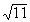}

 3a.  Natural numbers (answer/discussion to 3a) 3b.  Whole numbers (answer/discussion to 3b) 3c.  Integers (answer/discussion to 3c)

 3d.  Rational numbers (answer/discussion to 3d) 3e.  Irrational numbers (answer/discussion to 3e) 3f.  Real numbers (answer/discussion to 3f)Practice Problems 4a - 4c: Placeorto make each statement true.

 4a.  0  ? {x | x is a rational number} (answer/discussion to 4a) 4b.  10  ? { 2, 4, 6, 8, ...} (answer/discussion to 4b)

 4c.?  {x | x is an irrational number} (answer/discussion to 4c)Practice Problems 5a - 5b: Determine whether the statement is true or false.

 5a QR (answer/discussion to 5a) 5b.   RZ (answer/discussion to 5b)Practice Problems 6a - 6b: Find the absolute value.Practice Problems 7a - 7b: Write the opposite of the number.Need Extra Help on these Topics?

The following are webpages that can assist you in the topics that were covered on this page:

Go to Get Help Outside the Classroom found in Tutorial 1: How to Succeed in a Math Class for some more suggestions.Last revised on June 10, 2011 by Kim Seward.